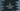# C program to find the nth fibonacci number# Introduction :

The Fibonacci series is a series of numbers where each value is the sum of its two preceding numbers. Each number in the series is called a Fibonacci number. By convention, the first and the second number of a Fibonacci series are defined as 0 and 1. For example : 0, 1, 1, 2, 3, 5, 8, 13, 21… is a Fibonacci series.

In this C programming tutorial, we will learn how to find the nth Fibonacci number. The program will take the value of n as an input from the user and it will print out the result to the user. We will find it using a recursive function.

## C program :

``````#include <stdio.h>

//3
int findFibonacci(int n)
{
//4
if (n == 1 || n == 0)
{
return n;
}

//5
return (findFibonacci(n - 1) + findFibonacci(n - 2));
}

int main(int argc, char const *argv[])
{
//1
int n;
printf("Enter the value of n :");
scanf("%d", &n);

//2
printf("%dth number : %d", n, findFibonacci(n - 1));
return 0;
}``````

## Explanation :

The commented numbers in the above program denote the step numbers below :

1. Ask the user to enter the value of n. Read it and store it in variable n.
2. Find the nth Fibonacci number using function findFibonacci.
3. findFibonacci takes one integer argument, finds the nth Fibonacci number and returns it.
4. If the value of n is 1 or 0, return it. i.e. for 0 return 0 and for 1 return 1.
5. Else, call the method findFibonacci recursively to find out the value.

### Sample Output :

``````Enter the value of n :7
7th number : 8``````